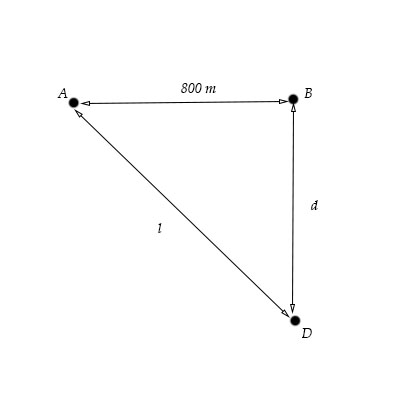# Consider a ship that travels along a straight line parallel to the shore and a distance d = 500 \...

## Question:

Consider a ship that travels along a straight line parallel to the shore and a distance {eq}d = 500 \ m {/eq} from it. The ship's radio receives simultaneous signals of the same frequency from antennas {eq}A {/eq} and {eq}B {/eq}, separated by a distance {eq}L = 800 \ m {/eq}. The signals interfere constructively at point {eq}C {/eq}, which is equidistant from {eq}A {/eq} and {eq}B {/eq}. The signal goes through the first minimum at point {eq}D {/eq}, which is directly outward from the shore from point {eq}B {/eq}. Determine the wavelength of the radio waves.## Sound Interference:

Sound waves are known to undergo interference, whether constructively or destructively, depending on their phase difference. This, in turn, is dependent upon their relative displacement from their respective sources.

In order for destructive interference to occur, the following condition must be satisfied:

{eq}\displaystyle l - d = \left( m + \frac{1}{2} \right) \lambda {/eq}

where {eq}l {/eq} and {eq}d {/eq} are distances as given in the figure, {eq}m {/eq} is the order of the minimum (m=1 for the first-order minimum), and {eq}\lambda {/eq} is the wavelength.

Here, we first solve for {eq}l {/eq} by applying the Pythagorean theorem:

{eq}\displaystyle l = \sqrt{ (800\ \rm m)^2 + d^2} = \sqrt{ (800\ \rm m)^2 + (500\ \rm m)^2} = \sqrt{ 890, 000\ \rm m^2 } = \sqrt{ 890, 000} \ \rm m {/eq}

Now, we wolve for the wavelength:

{eq}\displaystyle \begin{align} l - d &= \left( m + \frac{1}{2} \right) \lambda\\ \sqrt{ 890, 000} \ \rm m - 500\ \rm m &= \left( 1 + \frac{1}{2} \right) \lambda \\ 443.398\ \rm m &= \frac{3}{2} \lambda \\ \lambda & \approx 296\ \rm m \end{align} {/eq}